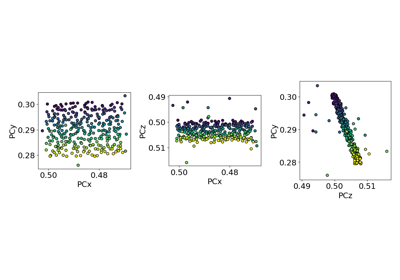# estimate_xtilt_ztilt#

EBSDDetector.estimate_xtilt_ztilt(degrees: bool = False, is_outlier: = None) [source]#

Estimate the tilts about the detector $$X_d$$ and $$Z_d$$ axes.

These tilts bring the sample plane normal into coincidence with the detector plane normal (but in the opposite direction) .

See the reference frame tutorial for details on the detector sample geometry.

Estimates are found by fitting a hyperplane to pc using singular value decomposition.

Parameters:
degrees

Whether to return the estimated tilts in radians (False, default) or degrees (True).

is_outlier

Boolean array with True for PCs to not include in the fit. If not given, all PCs are used. Must be of navigation_shape.

Returns:
x_tilt

Estimated tilt about detector $$X_d$$ in radians (degrees=False) or degrees (degrees=True).

z_tilt

Estimated tilt about detector $$Z_d$$ in radians (degrees=False) or degrees (degrees=True).

Notes

This method is adapted from Aimo Winkelmann’s function fit_plane() in the xcdskd Python package. Its use is described in .

Winkelmann refers to Gander & Hrebicek, “Solving Problems in Scientific Computing”, 3rd Ed., Chapter 6, p. 97 for the implementation of the hyperplane fitting.

## Examples using EBSDDetector.estimate_xtilt_ztilt#Estimate tilts about the detector x and z axis

Estimate tilts about the detector x and z axis